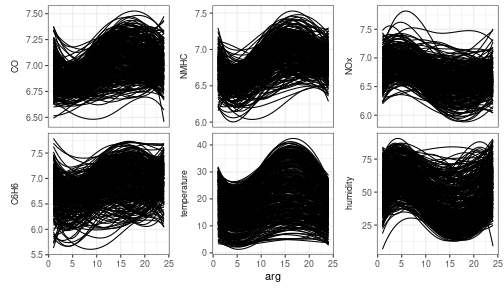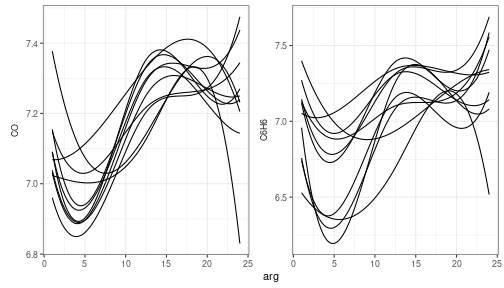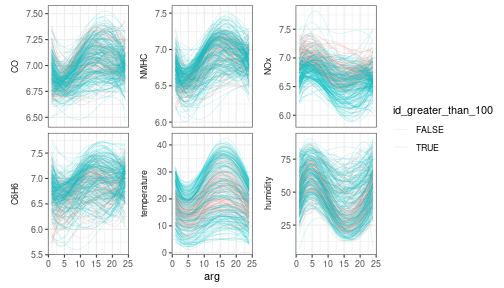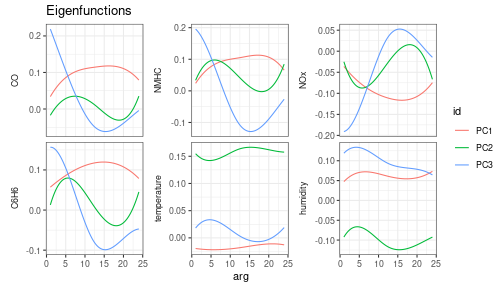# The mfd class

Let us show how the funcharts package works through an example with the dataset air, which has been included from the R package FRegSigCom and is used in the paper of Qi and Luo (2019).

NOTE: since the objective of this vignette is only to illustrate how the package works, in the following we will use only 5 basis functions and a fixed smoothing parameter to reduce the computational time.

## Getting multivariate functional data and the mfd class

We provide the mfd class for multivariate functional data. It inherits from the fd class but provides some additional features:

• It forces the coef argument to be an array even when the number of functional observations and/or the number of functional variables are one
• It provides a better subset function [ that never drops dimensions, then it always returns a mfd object with three-dimensional array argument coef; moreover it allows extracting observations/variables also by name
• When possible, it stores the original raw data in the long data frame format

The first thing is to get the mfd object from discrete data. We currently allow two types of input with the two functions:

1. get_mfd_data.frame: first input must be a data.frame in the long format, with:
• one arg column giving the argument (x) values,
• one id column indicating the functional observation,
• one column per each functional variable indicating the corresponding y values
2. get_mfd_list: first input must be a list of matrices for the case all functional data are observed on the same grid, which:
• must have all the same dimension,
• have the variable names as name of the list,
• are such that, for each matrix:
• each row corresponds to a functional observation
• each column corresponds to a point on the grid

In this example, the dataset air is in the second format (list of matrices, with data observed on the same grid)

library(funcharts)
data("air")
fun_covariates <- names(air)[names(air) != "NO2"]
mfdobj_x <- get_mfd_list(air[fun_covariates],
grid = 1:24,
n_basis = 5,
lambda = 1e-2)

In order to perform the statistical process monitoring analysis, we divide the dataset into a phase I and a phase II dataset.

rows1 <- 1:300
rows2 <- 301:355
mfdobj_x1 <- mfdobj_x[rows1]
mfdobj_x2 <- mfdobj_x[rows2]

Now we extract the scalar response variable, i.e. the mean of NO2 at each observation:

y <- rowMeans(air$NO2) y1 <- y[rows1] y2 <- y[rows2] ## Plotting functions We also provide plotting functions using ggplot2. plot_mfd(mfdobj_x1)plot_mfd(mfdobj_x1[1:10, c("CO", "C6H6")])This functions provides a layer geom_mfd, which is basically a geom_line that is added to ggplot() to plot functional data. It also allows to plot the original raw data by adding the argument type_mfd = "raw". geom_mfd accepts the argument data as input, which must be a data frame with two columns, id and var, in order to use aesthetic mappings that allow for example to colour different functions according to some columns in this data frame. dat <- data.frame(id = unique(mfdobj_x1$raw\$id)) %>%
mutate(id_greater_than_100 = as.numeric(id) > 100)
ggplot() +
geom_mfd(mapping = aes(col = id_greater_than_100),
mfdobj = mfdobj_x1,
data = dat,
alpha = .2,
lwd = .3)## Multivariate functional principal component analysis

For class mfd we provide a function pca_mfd, which is a wrapper to pca.fd. It returns multivariate functional principal component scores summed over variables (fda::pca.fd returns an array of scores when providing a multivariate functional data object). Moreover, the eigenfunctions or multivariate functional principal components given in harmonics argument are converted to the mfd class. We also provide a plot function for the eigenfunctions (the argument harm selects which components to plot).

pca <- pca_mfd(mfdobj_x1)
plot_pca_mfd(pca, harm = 1:3)